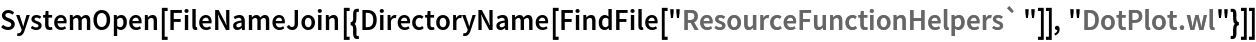Function Repository Resource:

# DotPlot

Create a dot plot for a set of real-valued univariate data

Contributed by: Wolfram|Alpha Math Team
 ResourceFunction["DotPlot"][data] plots the integer data on a number line, stacking repeated values.

## Details

ResourceFunction["DotPlot"] has the same options as NumberLinePlot.

## Examples

### Basic Examples (1)

Get a dot plot for a set of univariate data:

 In:=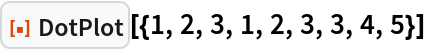Out=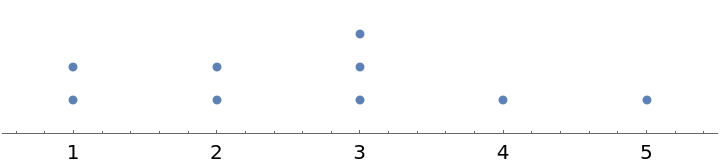### Scope (1)

Get a dot plot for a random dataset:

 In:=Out=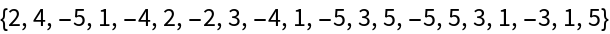Out=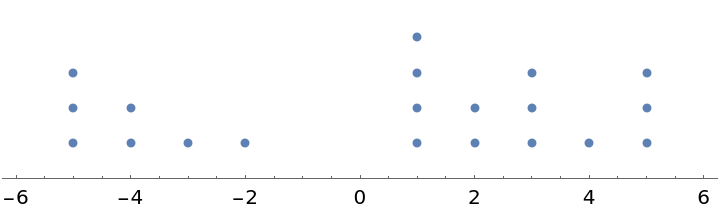### Options (1)

DotPlot has the same options as NumberLinePlot:

 In:=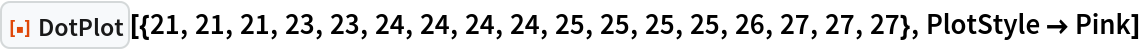Out=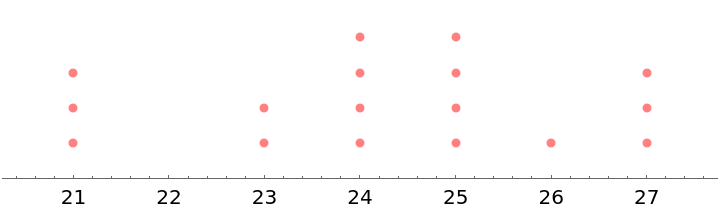### Possible Issues (1)

DotPlot only supports real-valued datasets:

 In:=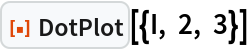Out=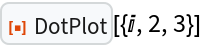## Publisher

Wolfram|Alpha Math Team

## Version History

• 2.0.0 – 23 March 2023
• 1.0.0 – 19 December 2022

## Author Notes

To view the full source code for DotPlot, evaluate the following: